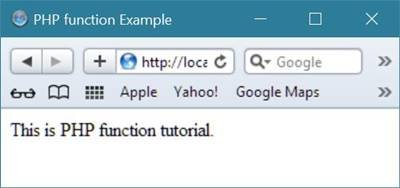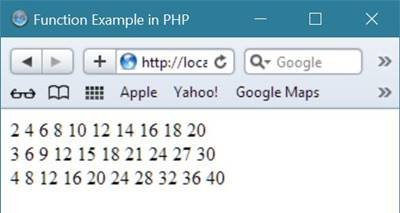# PHP Functions

The real power of PHP comes from its functions. There are more than about 1000 built-in functions available in PHP, which is much enough to perform a lot of task.

## PHP5 Functions

You are free to create your own functions (user-defined functions). A function is basically a block of code that can be used to perform some particular task in your program.

## Create a Function in PHP

A function in PHP is created with the help of function keyword. Here is the general form to declare a function in PHP:

```function functionName()
{
code to be executed here;
}```

Here, function is the keyword used in declaring the function in PHP, functionName is the name of the function. You can give a name to the function starting with a letter or underscore (but not with a number).

## PHP Functions Example

Here is an example, creates a function named writeMsg(), to write a message as output:

```<!DOCTYPE html>
<html>
<head>
<title>PHP function Example</title>
</head>
<body>
<?php
function writeMsg()
{
echo "This is PHP function tutorial.";
}
writeMsg();
?>
</body>
</html>```

Here is the output produced by the above PHP script:A function with parameters is known as parameterized function in PHP.

Here is another example, uses function with parameters in PHP:

```<html>
<head>
<title>Writing PHP Function with Parameters</title>
</head>
<body>
<?php
function addFunction(\$num1, \$num2)
{
\$sum = \$num1 + \$num2;
echo "Sum of the passed numbers is : \$sum";
}
addFunction(100, 200);
?>
</body>
</html>```

Here is the output of this PHP script:Let's take another example also demonstrating functions in PHP with parameter.

```<html>
<head>
<title>Function Example in PHP</title>
</head>
<body>
<?php
function printTable(\$num)
{
for(\$i=1;\$i<=10;\$i++)
{
echo \$num*\$i;
echo " ";
}
}
printTable(2);
echo "<br/>";
printTable(3);
echo "<br/>";
printTable(4);
echo "<br/>";
?>
</body>
</html>```

Here is the sample output produced by the above parameterized function in PHP example.Tools
Calculator

Quick Links
Signup - Login - Give Online Test